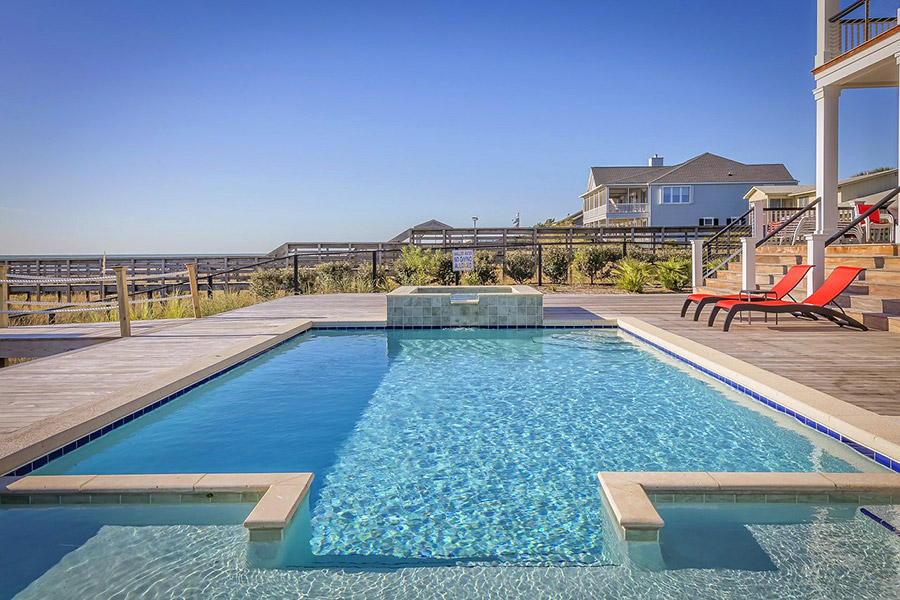# Pool Volume Calculator

Use this pool calculator to estimate how many gallons or liters of water you need to fill a swimming pool of a given size. Calculates the volume of the swimming pool and the volume of water required, as well as its weight. It can be used to estimate how much water is in your pool.

### Calculation results

0.444 cubic yards (12 ft3) Swimming pool volume Water needed Water weight
Share calculator:

Embed this tool:
get code

## Calculating pool volume and water needed

One often needs to calculate the volume of a swimming pool in order to determine the amount of water needed to fill it, usually as a part of an estimation for the cost of maintaining the pool, including the water cost and the amount of chlorine needed to disinfect it. Calculations for the weight of the water the swimming pool will contain are required when designing the building foundations for it, making sure they can hold the weight. This pool volume calculator works well for both occasions and the way to use it is by following this process:

1. Estimate the volume of water that will fill the pool by taking measurements or using dimensions specified in an architectural plan or product specifications.
2. Convert the volume to litres or gallons, depending on the unit you want the result to be in
3. To calculate the water weight, multiply the volume by its density (in the same units). The density of water is ~62.2347 lb/ft3 (997 kg/m3) at room temperature.

When using this pool calculator, you should consider that the depth of the pool is not the whole depth from top to bottom, but only the depth to which it can actually be filled without water overflowing into the drains. Some incorrectly refer to the volume of a swimming pool as "swimming pool size". However, "size" is the internal dimensions of the pool, not its volume, although the two are related.

## Volume and water calculations for irregularly-shaped swimming pools

In some cases, the swimming pool will have an irregular shape, for example the floor may be inclined or step-wise, or the outer walls may be curves instead of straight lines or a circle. However, our calculator only supports rectangular pools and circular pools.

In such cases what you can do is divide the volume in several approximately regularly-shaped sections, calculate their volume and water requirements and then sum them up together. In case you end up needing to do this for a large number of sections, you might use our summation calculator. Reasonable approximations can be made for slightly irregular shapes by taking the average length or width, but if you need a very accurate water estimation you should consult a professional. The math will likely involve integral calculus.## Pool Volume Calculation Examples

Example 1: Rectangular Pool. Let us say you need to fill a swimming pool with a simple rectangular shape such as a semi-olympic size with a length of 25 meters and width of 12.5 meters (compared to an Olympic swimming pool which is 50 meters by 25 meters). If it's depth is 2 meters all across, how much water do we need to fill it up?

Answer: This is a simple volume of a box calculation. The formula is Volume = Width · Height · Depth. In our case that's 25m x 12.5m x 2m = 25m x 25m2 = 625m3 so we need six hundred twenty five cubic meters of water.

Example 2: L-shaped pool. A slightly more complex shape would be if one has a swimming pool with an L-shape and wants to know how much water is in their pool. If the left-side outer edge of the L has a length of 25 yards, the bottom of the L has a length of 15 yards and the right-side of the L has a length of 5 yards, and the top side has a length of 10 yards how do we find the total pool volume in cubic yards if we also know the depth is 2.5 yards?

Answer: First we split the L into two rectangular boxes, one with sides of 25 yards and 10 yards, and another with 15-10 = 5 and 5 yards. We calculate the two volumes separately: volume 1 is 25yd x 10yd x 2.5yd = 625 cu yd and volume 2 is 5yd x 5yd x 2.5yd = 62.5 cu yd. Then we simply sum the two parts of the pool: 625yd3 + 62.5yd3 = 687.5yd3.

### How much water is in my pool?

To calculate how many gallons does a pool contain, assume the same L-shaped pool from "Example 2" above. It is also known that 1 cubic yard contains approximately 201.974 gallons of water.

Example 3: How many gallons of water are in my pool?

Answer: It has already been calculated that the volume of the pool is 687.5yd3. Multiply the pool volume by 201.974 to get the number of gallons the pool contains when full: 687.5 x 201.974 = 138,857.125 gallons.

### Liter vs litre

Both "liter" and "litre" refer to the same unit, with "liter" being used in the United States while the conventional name as defined by the international body of standardization is "litre". Since they express the same volume of gas or liquid, the two names can be used interchangeably which is what we do in this calculator.

#### Cite this calculator & page

If you'd like to cite this online calculator resource and information as provided on the page, you can use the following citation:
Georgiev G.Z., "Pool Volume Calculator", [online] Available at: https://www.gigacalculator.com/calculators/pool-volume-calculator.php URL [Accessed Date: 01 Apr, 2023].